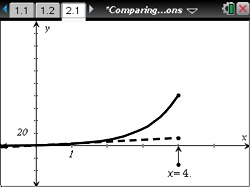•##### Download

•• ##### Device
•TI-Nspire™ CX/CX II
•TI-Nspire™ CX CAS/CX II CAS
• TI-Nspire™
• TI-Nspire™ CAS
• ##### Software

TI-Nspire™
TI-Nspire™ CAS

4.5

• ##### Report an Issue

Algebra 1: Comparing Linear and Exponential Functions
by Texas Instruments

Updated on August 01, 2019#### Objectives

• Use a table and a graph to compare the changes in linear and exponential expressions as x is increased
• Recognize that as x increases, a linear expression increases at a constant rate (additively) while an exponential function increases multiplicatively

#### Vocabulary

• Linear expression
• Exponential expression

#### About the Lesson

This lesson involves comparing data from two different scenarios—linear and exponential growth. As a result, students will:

• Complete a table involving prize money scenarios.
• Examine the table for trends in the growth of each prize money scenarios.
• Examine the graphs to compare the data.